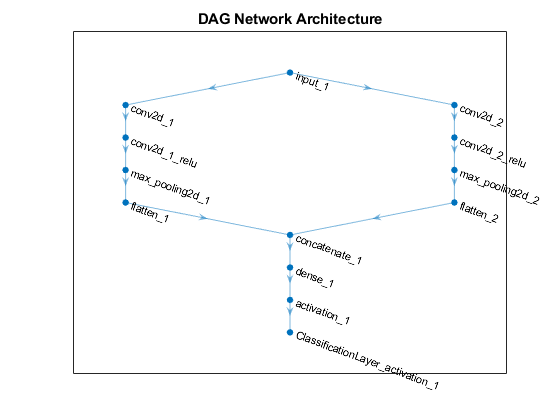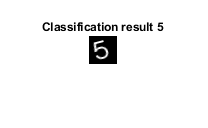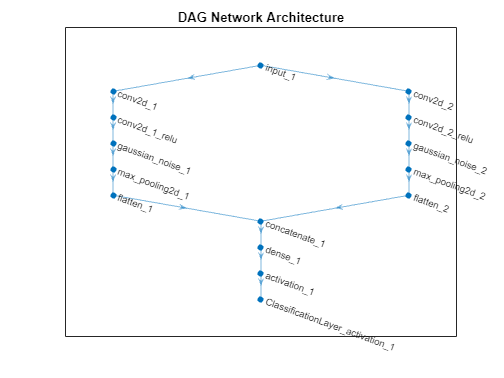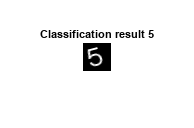# importTensorFlowNetwork

Import pretrained TensorFlow network

## Syntax

``net = importTensorFlowNetwork(modelFolder)``
``net = importTensorFlowNetwork(modelFolder,Name,Value)``

## Description

example

````net = importTensorFlowNetwork(modelFolder)` imports a pretrained TensorFlow™ network from the folder `modelFolder`, which contains the model in the saved model format (compatible only with TensorFlow 2). The function can import TensorFlow networks created with the TensorFlow-Keras sequential or functional API. `importTensorFlowNetwork` imports the layers defined in the `saved_model.pb` file and the learned weights contained in the `variables` subfolder, and returns the network `net` as a `DAGNetwork` or `dlnetwork` object. `importTensorFlowNetwork` requires the Deep Learning Toolbox™ Converter for TensorFlow Models support package. If this support package is not installed, then `importTensorFlowNetwork` provides a download link. Note`importTensorFlowNetwork` tries to generate a custom layer when you import a custom TensorFlow layer or when the software cannot convert a TensorFlow layer into an equivalent built-in MATLAB® layer. For a list of layers for which the software supports conversion, see TensorFlow-Keras Layers Supported for Conversion into Built-In MATLAB Layers. `importTensorFlowNetwork` saves the generated custom layers and the associated TensorFlow operators in the package `+modelFolder`. `importTensorFlowNetwork` does not automatically generate a custom layer for each TensorFlow layer that is not supported for conversion into a built-in MATLAB layer. For more information on how to handle unsupported layers, see Tips. ```

example

````net = importTensorFlowNetwork(modelFolder,Name,Value)` imports the pretrained TensorFlow network with additional options specified by one or more name-value arguments. For example, `'OutputLayerType','classification'` imports the network as a `DAGNetwork` with a classification output layer appended to the end of the imported network architecture.```

## Examples

collapse all

Import a pretrained TensorFlow network in the saved model format as a `DAGNetwork` object, and use the imported network to classify an image.

Specify the model folder.

```if ~exist('digitsDAGnet','dir') unzip('digitsDAGnet.zip') end modelFolder = './digitsDAGnet';```

Specify the class names.

`classNames = {'0','1','2','3','4','5','6','7','8','9'};`

Import a TensorFlow network in the saved model format. By default, `importTensorFlowNetwork` imports the network as a `DAGNetwork` object. Specify the output layer type for an image classification problem.

`net = importTensorFlowNetwork(modelFolder,'OutputLayerType','classification','Classes',classNames)`
```Importing the saved model... Translating the model, this may take a few minutes... Finished translation. Assembling network... Import finished. ```
```net = DAGNetwork with properties: Layers: [13×1 nnet.cnn.layer.Layer] Connections: [13×2 table] InputNames: {'input_1'} OutputNames: {'ClassificationLayer_activation_1'} ```

Plot the network architecture.

```plot(net) title('DAG Network Architecture')```Read the image you want to classify and display the size of the image. The image is a grayscale (one-channel) image with size 28-by-28 pixels.

```digitDatasetPath = fullfile(toolboxdir('nnet'),'nndemos','nndatasets','DigitDataset'); I = imread(fullfile(digitDatasetPath,'5','image4009.png')); size(I)```
```ans = 1×2 28 28 ```

Display the input size of the network. In this case, the image size matches the network input size. If they do not match, you must resize the image by using `imresize(I, netInputSize(1:2))`.

`net.Layers(1).InputSize`
```ans = 1×3 28 28 1 ```

Classify the image using the pretrained network.

`label = classify(net,I);`

Display the image and the classification result.

```imshow(I) title(['Classification result ' char(label)])```Import a pretrained TensorFlow Network in the saved model format as a `dlnetwork` object, and use the imported network to predict class labels.

Specify the model folder.

```if ~exist('digitsDAGnet','dir') unzip('digitsDAGnet.zip') end modelFolder = './digitsDAGnet';```

Specify the class names.

`classNames = {'0','1','2','3','4','5','6','7','8','9'};`

Import a TensorFlow network in the saved model format as a `dlnetwork` object.

`net = importTensorFlowNetwork(modelFolder,'TargetNetwork','dlnetwork')`
```Importing the saved model... Translating the model, this may take a few minutes... Finished translation. Assembling network... Import finished. ```
```net = dlnetwork with properties: Layers: [12×1 nnet.cnn.layer.Layer] Connections: [12×2 table] Learnables: [6×3 table] State: [0×3 table] InputNames: {'input_1'} OutputNames: {'activation_1'} Initialized: 1 ```

Read the image you want to classify and display the size of the image. The image is a grayscale (one-channel) image with size 28-by-28 pixels.

```digitDatasetPath = fullfile(toolboxdir('nnet'),'nndemos','nndatasets','DigitDataset'); I = imread(fullfile(digitDatasetPath,'5','image4009.png')); size(I)```
```ans = 1×2 28 28 ```

Display the input size of the network. In this case, the image size matches the network input size. If they do not match, you must resize the image by using `imresize(I, netInputSize(1:2))`.

`netInputSize = net.Layers(1).InputSize`
```netInputSize = 1×3 28 28 1 ```

Convert the image to a `dlarray`. Format the images with the dimensions `'SSCB'` (spatial, spatial, channel, batch). In this case, the batch size is 1 and you can omit it (`'SSC'`).

`I_dlarray = dlarray(single(I),'SSCB');`

Classify the sample image and find the predicted label.

```prob = predict(net,I_dlarray); [~,label] = max(prob);```

Display the image and the classification result.

```imshow(I) title(['Classification result ' classNames{label}]) ```Import a pretrained TensorFlow network in the saved model format as a `DAGNetwork` object, and use the imported network to classify an image. The imported network contains layers that are not supported for conversion into built-in MATLAB layers. The software automatically generates custom layers when you import these layers.

This example uses the helper function `findCustomLayers`. To view the code for this function, see Helper Function.

Specify the model folder.

```if ~exist('digitsDAGnetwithnoise','dir') unzip('digitsDAGnetwithnoise.zip') end modelFolder = './digitsDAGnetwithnoise';```

Specify the class names.

`classNames = {'0','1','2','3','4','5','6','7','8','9'};`

Import a TensorFlow network in the saved model format. By default, `importTensorFlowNetwork` imports the network as a `DAGNetwork` object. Specify the output layer type for an image classification problem.

`net = importTensorFlowNetwork(modelFolder,'OutputLayerType','classification','Classes',classNames);`
```Importing the saved model... Translating the model, this may take a few minutes... Finished translation. Assembling network... Import finished. ```

If the imported network contains layers not supported for conversion into built-in MATLAB layers, then `importTensorFlowNetwork` can automatically generate custom layers in place of these layers. `importTensorFlowNetwork` saves each generated custom layer to a separate `.m` file in the package `+digitsDAGnetwithnoise` in the current folder.

Find the indices of the automatically generated custom layers using the helper function `findCustomLayers`, and display the custom layers.

```ind = findCustomLayers(net.Layers,'+digitsDAGnetwithnoise'); net.Layers(ind)```
```ans = 2×1 Layer array with layers: 1 'gaussian_noise_1' GaussianNoise digitsDAGnetwithnoise.kGaussianNoise1Layer3766 2 'gaussian_noise_2' GaussianNoise digitsDAGnetwithnoise.kGaussianNoise2Layer3791 ```

Plot the network architecture.

```plot(net) title('DAG Network Architecture')```Read the image you want to classify.

```digitDatasetPath = fullfile(toolboxdir('nnet'),'nndemos','nndatasets','DigitDataset'); I = imread(fullfile(digitDatasetPath,'5','image4009.png'));```

Classify the image using the pretrained network.

`label = classify(net,I);`

Display the image and the classification result.

```imshow(I) title(['Classification result ' char(label)])```Helper Function

This section provides the code of the helper function `findCustomLayers` used in this example. `findCustomLayers` returns the `indices` of the custom layers that `importTensorFlowNetwork` automatically generates.

```function indices = findCustomLayers(layers,PackageName) s = what(['.\' PackageName]); indices = zeros(1,length(s.m)); for i = 1:length(layers) for j = 1:length(s.m) if strcmpi(class(layers(i)),[PackageName(2:end) '.' s.m{j}(1:end-2)]) indices(j) = i; end end end end```

## Input Arguments

collapse all

Name of the folder containing the TensorFlow model, specified as a character vector or string scalar. `modelFolder` must be in the current folder, or you must include a full or relative path to the folder. `modelFolder` must contain the file `saved_model.pb`, and the subfolder `variables`. It can also contain the subfolders `assets` and `assets.extra`.

• The file `saved_model.pb` contains the layer graph architecture and training options (for example, optimizer, losses, and metrics).

• The subfolder `variables` contains the weights learned by the pretrained TensorFlow network. By default, `importTensorFlowNetwork` imports the weights.

• The subfolder `assets` contains supplementary files (for example, vocabularies), which the layer graph can use. `importTensorFlowNetwork` does not import the files in `assets`.

• The subfolder `assets.extra` contains supplementary files (for example, information for users), which coexist with the layer graph.

Example: `'MobileNet'`

Example: `'./MobileNet'`

### Name-Value Arguments

Specify optional pairs of arguments as `Name1=Value1,...,NameN=ValueN`, where `Name` is the argument name and `Value` is the corresponding value. Name-value arguments must appear after other arguments, but the order of the pairs does not matter.

Before R2021a, use commas to separate each name and value, and enclose `Name` in quotes.

Example: `importTensorFlowNetwork(modelFolder,'TargetNetwork','dagnetwork','OutputLayerType','classification')` imports a network from `modelFolder` as a `DAGNetwork` object, saves the automatically generated custom layers in the package `+modelFolder` in the current folder, and appends a classification output layer to the end of the imported network architecture.

Name of the package in which `importTensorFlowNetwork` saves custom layers, specified as a character vector or string scalar. `importTensorFlowNetwork` saves the custom layers package `+PackageName` in the current folder. If you do not specify `'PackageName'`, then `importTensorFlowNetwork` saves the custom layers in a package named `+modelFolder` in the current folder. For more information on packages, see Packages Create Namespaces.

`importTensorFlowNetwork` tries to generate a custom layer when you import a custom TensorFlow layer or when the software cannot convert a TensorFlow layer into an equivalent built-in MATLAB layer. `importTensorFlowNetwork` saves each generated custom layer to a separate `.m` file in `+PackageName`. To view or edit a custom layer, open the associated `.m` file. For more information on custom layers, see Deep Learning Custom Layers.

The package `+PackageName` can also contain the subpackage `+ops`. This subpackage contains MATLAB functions corresponding to TensorFlow operators (see Supported TensorFlow Operators) that are used in the automatically generated custom layers. `importTensorFlowNetwork` saves the associated MATLAB function for each operator in a separate `.m` file in the subpackage `+ops`. The object functions of `dlnetwork`, such as the `predict` function, use these operators when interacting with the custom layers.

Example: `'PackageName','MobileNet'`

Example: `'PackageName','CustomLayers'`

Target type of Deep Learning Toolbox network, specified as `'dagnetwork'` or `'dlnetwork'`.

• Specify `'TargetNetwork` as `'dagnetwork'` to import the network as a `DAGNetwork` object. In this case, `net` must include an output layer specified by the TensorFlow saved model loss function or the name-value argument `'OutputLayerType'`.

• Specify `'TargetNetwork` as `'dlnetwork'` to import the network as a `dlnetwork` object. In this case, `net` does not include an output layer.

Example: `'TargetNetwork','dlnetwork'`

Type of output layer that `importTensorFlowNetwork` appends to the end of the imported network architecture, specified as `'classification'`, `'regression'`, or `'pixelclassification'`. Appending a `pixelClassificationLayer` (Computer Vision Toolbox) object requires Computer Vision Toolbox™.

• If you specify `'TargetNetwork'` as `'dagnetwork'` and the saved model in `modelFolder` does not specify a loss function, you must assign a value to the name-value argument `'OutputLayerType'`. A `DAGNetwork` object must have an output layer.

• If you specify `'TargetNetwork'` as `'dlnetwork'`, `importTensorFlowNetwork` ignores the name-value argument `'OutputLayerType'`. A `dlnetwork` object does not have an output layer.

Example: `'OutputLayerType','classification'`

Size of the input images for the network, specified as a vector of two or three numerical values corresponding to `[height,width]` for grayscale images and `[height,width,channels]` for color images, respectively. The network uses this information when the `saved_model.pb` file in `modelFolder` does not specify the input size.

Example: `'ImageInputSize',[28 28]`

Classes of the output layer, specified as a categorical vector, string array, cell array of character vectors, or `'auto'`. If you specify a string array or cell array of character vectors `str`, then `importTensorFlowNetwork` sets the classes of the output layer to `categorical(str,str)`. If `Classes` is `'auto'`, then `importTensorFlowNetwork` sets the classes to `categorical(1:N)`, where `N` is the number of classes.

• If you specify `'TargetNetwork'` as `'dagnetwork'`, `importTensorFlowNetwork` stores information on classes in the output layer of the `DAGNetwork` object.

• If you specify `'TargetNetwork'` as `'dlnetwork'`, `importTensorFlowNetwork` ignores the name-value argument `'Classes'`. A `dlnetwork` object does not have an output layer to store information on classes.

Example: `'Classes',{'0','1','3'}`

Example: `'Classes',categorical({'dog','cat'})`

Data Types: `char` | `categorical` | `string` | `cell`

Indicator to display import progress information in the command window, specified as a numeric or logical `1` (`true`) or `0` (`false`).

Example: `'Verbose','true'`

## Output Arguments

collapse all

Pretrained TensorFlow network, returned as a `DAGNetwork` or `dlnetwork` object.

• Specify `'TargetNetwork` as `'dagnetwork'` to import the network as a `DAGNetwork` object. On the `DAGNetwork` object, you then predict class labels by using the `classify` function.

• Specify `'TargetNetwork` as `'dlnetwork'` to import the network as a `dlnetwork` object. On the `dlnetwork` object, you then predict class labels by using the `predict` function. Specify the input data as a `dlarray` using the correct data format (for more information, see the `fmt` argument of `dlarray`).

## Limitations

• `importTensorFlowNetwork` supports TensorFlow versions v2.0 to 2.6.

collapse all

### TensorFlow-Keras Layers Supported for Conversion into Built-In MATLAB Layers

`importTensorFlowNetwork` supports the following TensorFlow-Keras layer types for conversion into built-in MATLAB layers, with some limitations.

TensorFlow-Keras LayerCorresponding Deep Learning Toolbox Layer
`Add``additionLayer`

`Activation`, with activation names:

• `elu`

• `gelu`

• `relu`

• `linear`

• `softmax`

• `sigmoid`

• `swish`

• `tanh`

Layers:

• `ELU`

• `Softmax`

• `ReLU`

• `LeakyReLU`

• `PReLu`*

Layers:

`AveragePooling1D``averagePooling1dLayer` with `PaddingValue` specified as `'mean'`
`AveragePooling2D``averagePooling2dLayer` with `PaddingValue` specified as `'mean'`
`BatchNormalization``batchNormalizationLayer`
`Bidirectional(LSTM(__))``bilstmLayer`
`Concatenate``depthConcatenationLayer`
`Conv1D``convolution1dLayer`
`Conv2D``convolution2dLayer`
`Conv2DTranspose``transposedConv2dLayer`
`CuDNNGRU``gruLayer`
`CuDNNLSTM``lstmLayer`
`Dense``fullyConnectedLayer`
`DepthwiseConv2D``groupedConvolution2dLayer`
`Dropout``dropoutLayer`
`Embedding``wordEmbeddingLayer` (Text Analytics Toolbox)
`Flatten``nnet.keras.layer.FlattenCStyleLayer`
`GlobalAveragePooling1D``globalAveragePooling1dLayer`
`GlobalAveragePooling2D``globalAveragePooling2dLayer`
`GlobalMaxPool1D``globalMaxPooling1dLayer`
`GlobalMaxPool2D``globalMaxPooling2dLayer`
`GRU``gruLayer`
`Input``imageInputLayer`, `sequenceInputLayer`, or `featureInputLayer`
`LSTM``lstmLayer`
`MaxPool1D``maxPooling1dLayer`
`MaxPool2D``maxPooling2dLayer`
`Multiply``multiplicationLayer`
`SeparableConv2D``groupedConvolution2dLayer` or `convolution2dLayer`
`TimeDistributed``sequenceFoldingLayer` before the wrapped layer, and `sequenceUnfoldingLayer` after the wrapped layer
`UpSampling2D``resize2dLayer` (Image Processing Toolbox)
`UpSampling3D``resize3dLayer` (Image Processing Toolbox)
`ZeroPadding1D``nnet.keras.layer.ZeroPadding1DLayer`
`ZeroPadding2D``nnet.keras.layer.ZeroPadding2DLayer`

* For a PReLU layer, `importTensorFlowNetwork` replaces a vector-valued scaling parameter with the average of the vector elements. You can change the parameter back to a vector after import. For an example, see Import Keras PReLU Layer.

### Supported TensorFlow-Keras Loss Functions

`importTensorFlowNetwork` supports the following Keras loss functions:

• `mean_squared_error`

• `categorical_crossentropy`

• `sparse_categorical_crossentropy`

• `binary_crossentropy`

### Supported TensorFlow Operators

`importTensorFlowNetwork` supports the following TensorFlow operators for conversion into MATLAB functions with `dlarray` support.

TensorFlow OperatorCorresponding MATLAB Function
`Add``tfAdd`
`AddN``tfAddN`
`AddV2``tfAdd`
`Assert``assert`
`AvgPool``tfAvgPool`
`BatchMatMulV2``tfBatchMatMulV2`
`BiasAdd``tfBiasAdd`
`BroadcastTo``tfBroadcastTo`
`Cast``tfCast`
`ConcatV2``tfCat`
`Const`None (translated to weights in custom layer)
`Conv2D``tfConv2D`
`DepthToSpace``depthToSpace` (Image Processing Toolbox)
`DepthwiseConv2dNative``tfDepthwiseConv2D`
`Exp``exp`
`ExpandDims``tfExpandDims`
`FusedBatchNormV3``tfBatchnorm`
`GatherV2``tfGather`
`GreaterEqual``ge`, `>=`
`Identity`None (translated to value assignment in custom layer)
`IdentityN``tfIdentityN`
`L2Loss``tfL2Loss`
`LeakyRelu``leakyrelu`
`Less``lt`, `<`
`Log``log`
`MatMul``tfMatMul`
`MaxPool``tfMaxPool`
`Maximum``tfMaximum`
`Mean``tfMean`
`Minimum``tfMinimum`
`MirrorPad``tfMirrorPad`
`Mul``tfMul`
`Neg``minus`, `-`
`Pack``tfStack`
`Pad``tfPad`
`PadV2``tfPad`
`PartitionedCall`None (translated to function in custom layer methods)
`Pow``power`, `.^`
`Prod``tfProd`
`RandomStandardNormal``tfRandomStandardNormal`
`Range``tfRange`
`ReadVariableOp`None (translated to value assignment in custom layer)
`RealDiv``tfDiv`
`Relu``relu`
`Relu6``relu` and `min`
`Reshape``tfReshape`
`ResizeNearestNeighbor``dlresize` (Image Processing Toolbox)
`Rsqrt``sqrt`
`Shape``tfShape`
`Sigmoid``sigmoid`
`Size``tfSize`
`Softmax``softmax`
`SpaceToDepth``spaceToDepth` (Image Processing Toolbox)
`Square``.^2`
`Sqrt``sqrt`
`SquaredDifference``tfMul` or `tfSub`
`Squeeze``tfSqueeze`
`StatefulPartitionedCall`None (translated to function in custom layer methods)
`StopGradient``tfStopGradient`
`StridedSlice``tfStridedSlice` or `tfSqueeze`
`Sub``tfSub`
`Tanh``tanh`
`Tile``tfTile`
`Transpose``tfTranspose`

For more information on functions that operate on `dlarray` objects, see List of Functions with dlarray Support.

### Generate Code for Imported Network

You can use MATLAB Coder™ or GPU Coder™ together with Deep Learning Toolbox to generate MEX, standalone CPU, CUDA® MEX, or standalone CUDA code for an imported network. For more information, see Deep Learning Code Generation.

• Use MATLAB Coder with Deep Learning Toolbox to generate MEX or standalone CPU code that runs on desktop or embedded targets. You can deploy generated standalone code that uses the Intel® MKL-DNN library or the ARM® Compute library. Alternatively, you can generate generic C or C++ code that does not call third-party library functions. For more information, see Deep Learning with MATLAB Coder (MATLAB Coder).

• Use GPU Coder with Deep Learning Toolbox to generate CUDA MEX or standalone CUDA code that runs on desktop or embedded targets. You can deploy generated standalone CUDA code that uses the CUDA deep neural network library (cuDNN), the TensorRT™ high performance inference library, or the ARM Compute library for Mali GPU. For more information, see Deep Learning with GPU Coder (GPU Coder).

`importTensorFlowNetwork` returns the network `net` as a `DAGNetwork` or `dlnetwork` object. Both these objects support code generation. For more information on MATLAB Coder and GPU Coder support for Deep Learning Toolbox objects, see Supported Classes (MATLAB Coder) and Supported Classes (GPU Coder), respectively.

You can generate code for any imported network whose layers support code generation. For lists of the layers that support code generation with MATLAB Coder and GPU Coder, see Supported Layers (MATLAB Coder) and Supported Layers (GPU Coder), respectively. For more information on the code generation capabilities and limitations of each built-in MATLAB layer, see the Extended Capabilities section of the layer. For example, see Code Generation and GPU Code Generation of `imageInputLayer`.

### Use Imported Network on GPU

`importTensorFlowNetwork` does not execute on a GPU. However, `importTensorFlowNetwork` imports a pretrained neural network for deep learning as a `DAGNetwork` or `dlnetwork` object, which you can use on a GPU.

• If you import the network as a `DAGNetwork` object, you can make predictions with the imported network on either a CPU or GPU by using `classify`. Specify the hardware requirements using the name-value argument `ExecutionEnvironment`. For networks with multiple outputs, use the `predict` function for `DAGNetwork` objects.

• If you import the network as a `DAGNetwork` object, you can make predictions with the imported network on either a CPU or GPU by using `predict`. Specify the hardware requirements using the name-value argument `ExecutionEnvironment`. If the network has multiple outputs, specify the name-value argument `ReturnCategorical` as `true`.

• If you import the network as a `dlnetwork` object, you can make predictions with the imported network on either a CPU or GPU by using `predict`. The function `predict` executes on the GPU if either the input data or network parameters are stored on the GPU.

• If you use `minibatchqueue` to process and manage the mini-batches of input data, the `minibatchqueue` object converts the output to a GPU array by default if a GPU is available.

• Use `dlupdate` to convert the learnable parameters of a `dlnetwork` object to GPU arrays.

`net = dlupdate(@gpuArray,net)`

• You can train the imported network on either a CPU or GPU by using `trainNetwork`. To specify training options, including options for the execution environment, use the `trainingOptions` function. Specify the hardware requirements using the name-value argument `ExecutionEnvironment`. For more information on how to accelerate training, see Scale Up Deep Learning in Parallel, on GPUs, and in the Cloud.

Using a GPU requires Parallel Computing Toolbox™ and a supported GPU device. For information on supported devices, see GPU Computing Requirements (Parallel Computing Toolbox).

## Tips

• If the imported network contains a layer not supported for conversion into a built-in MATLAB layer (see TensorFlow-Keras Layers Supported for Conversion into Built-In MATLAB Layers) and `importTensorFlowNetwork` does not generate a custom layer, then `importTensorFlowNetwork` returns an error. In this case, you can still use `importTensorFlowLayers` to import the network architecture.

• To use a pretrained network for prediction or transfer learning on new images, you must preprocess your images in the same way the images that were used to train the imported model were preprocessed. The most common preprocessing steps are resizing images, subtracting image average values, and converting the images from BGR format to RGB format.

• To resize images, use `imresize`. For example, `imresize(image,[227,227,3])`.

• To convert images from RGB to BGR format, use `flip`. For example, `flip(image,3)`.

For more information on preprocessing images for training and prediction, see Preprocess Images for Deep Learning.

• The members of the package `+PackageName` (custom layers and TensorFlow operators) are not accessible if the package parent folder is not on the MATLAB path. For more information, see Packages and the MATLAB Path.

• MATLAB uses one-based indexing, whereas Python® uses zero-based indexing. In other words, the first element in an array has an index of 1 and 0 in MATLAB and Python, respectively. For more information on MATLAB indexing, see Array Indexing. In MATLAB, to use an array of indices (`ind`) created in Python, convert the array to `ind+1`.

• For more tips, see Tips on Importing Models from TensorFlow, PyTorch, and ONNX.

## Alternative Functionality

Use `importTensorFlowNetwork` or `importTensorFlowLayers` to import a TensorFlow network in the saved model format . Alternatively, if the network is in HDF5 or JSON format, use `importKerasNetwork` or `importKerasLayers` to import the network.

 Using the SavedModel format. https://www.tensorflow.org/guide/saved_model.